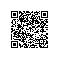# 用TensorFlow为图片添加字幕• 在获取图片里的重要信息时，我们如何基于图像分类模型的成功结果？

• 我们的模型如何能学习去融合对于语言的理解和对于图片的理解？

def get_data(annotation_path, feature_path):def build_model(self):

# declaring the placeholders for our extracted image feature vectors, our caption, and our mask

# (describes how long our caption is with an array of 0/1 values of length maxlen

img = tf.placeholder(tf.float32, [self.batch_size, self.dim_in])

caption_placeholder = tf.placeholder(tf.int32, [self.batch_size, self.n_lstm_steps])

# getting an initial LSTM embedding from our image_imbedding

image_embedding = tf.matmul(img, self.img_embedding) + self.img_embedding_bias

# setting initial state of our LSTM

state = self.lstm.zero_state(self.batch_size, dtype=tf.float32)

total_ loss = 0.0

with tf.variable_scope(“RNN”):

for i in range(self.n_lstm_steps):

if i > 0:

#if this isn’t the first iteration of our LSTM we need to get the word_embedding corresponding

# to the (i-1)th word in our caption

with tf.device(“/cpu:0”):

current_embedding = tf.nn.embedding_lookup(self.word_embedding, caption_placeholder[:,i-1]) + self.embedding_bias

else:

#if this is the first iteration of our LSTM we utilize the embedded image as our input

current_embedding = image_embedding

if i > 0:

# allows us to reuse the LSTM tensor variable on each iteration

tf.get_variable_scope().reuse_variables()

out, state = self.lstm(current_embedding, state)

print (out,self.word_encoding,self.word_encoding_bias)

if i > 0:

#get the one-hot representation of the next word in our caption

labels = tf.expand_dims(caption_placeholder[:, i], 1)

ix_range=tf.range(0, self.batch_size, 1)

ixs = tf.expand_dims(ix_range, 1)

concat = tf.concat([ixs, labels],1)

onehot = tf.sparse_to_dense(

concat, tf.stack([self.batch_size, self.n_words]), 1.0, 0.0)

#perform a softmax classification to generate the next word in the caption

logit = tf.matmul(out, self.word_encoding) + self.word_encoding_bias

xentropy = tf.nn.softmax_cross_entropy_with_logits(logits=logit, labels=onehot)

loss = tf.reduce_sum(xentropy)

total_loss += loss

def build_generator(self, maxlen, batchsize=1):

#same setup as build_model function

img = tf.placeholder(tf.float32, [self.batch_size, self.dim_in])

image_embedding = tf.matmul(img, self.img_embedding) + self.img_embedding_bias

state = self.lstm.zero_state(batchsize,dtype=tf.float32)

#declare list to hold the words of our generated captions

all_words = []

print (state,image_embedding,img)

with tf.variable_scope(“RNN”):

# in the first iteration we have no previous word, so we directly pass in the image embedding

# and set the previous_word to the embedding of the start token () for the future iterations

output, state = self.lstm(image_embedding, state)

previous_word = tf.nn.embedding_lookup(self.word_embedding, ) + self.embedding_bias

for i in range(maxlen):

tf.get_variable_scope().reuse_variables()

out, state = self.lstm(previous_word, state)

# get a one-hot word encoding from the output of the LSTM

logit = tf.matmul(out, self.word_encoding) + self.word_encoding_bias

best_word = tf.argmax(logit, 1)

with tf.device(“/cpu:0”):

# get the embedding of the best_word to use as input to the next iteration of our LSTM

previous_word = tf.nn.embedding_lookup(self.word_embedding, best_word)

previous_word += self.embedding_bias

all_words.append(best_word)

return img, all_words使用钉钉扫一扫加入圈子
+ 订阅